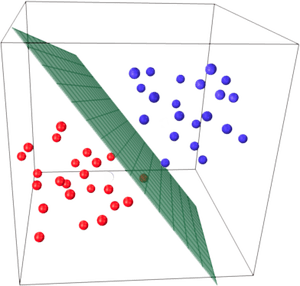## Logistic Regression Explained

Logistic regression is a pretty simple—yet very powerful—algorithm used in data science and machine learning. It is a statistical algorithm that classifies data by considering outcome variables on extreme ends and creates a logarithmic line to distinguish between them.

In the typical case, you’re trying to decide for a given data point whether a predicate is true or false. For example, if the data point is a credit card transaction, the predicate might be “this is a valid transaction” versus “this is a fraudulent transaction.” If the data point is a blood test, the predicate might be “this person will react well to this drug” versus “this person will react adversely.” And if the data point is an email message, the predicate might be “this is a real message” versus “this is spam.”

We always start with examples of data points, called training examples. If the predicate is true for an example, we say it’s a positive example; when the predicate is false, it’s a negative example.

The attributes of a given data point are represented numerically and can be imagined as a geometric point in space, like the picture below, where positive (blue) examples are geometrically different from negative (red) examples.

The whole game is then:

1) Learn how to geometrically distinguish positive examples from negative examples when given a training set of data points.

2) After you’ve learned, you can make a prediction on a new, as-yet-unseen data point. You just see which of the two groups of points the new point belongs in.(Source.)

So in this picture of training examples, blue points might each represent a valid credit card transaction, and red points might represent a fraudulent transaction.

1) The learning part of the game is to find a placement for the green plate in the picture that best splits the positive and negative examples in space. This is done mathematically, by computing the orientation and position in space for the green sheet relative to the example points so as to best separate the two groups.

Once you’ve placed the green sheet, we can say that positive examples always fall on one side of the sheet and negative examples fall on the other side.  And even better, our confidence in, say, the validity for a given credit transaction is just how far into valid territory the point is: blue points far from the green sheet on the are more confidently valid.

2) The prediction part then just takes a new, unseen point and asks (a) which side of the green sheet is this point and (b) how deep on that side is it? This gives us the true or false prediction and a sense of how confident we are in that prediction.

In logistic regression, the result of the prediction is always represented as a number between 0 and 1, where 0 means the predicate is false for a data point, 1 means the predicate is true, and 0.5 means we can’t really say, which is just when the point falls on the green sheet.

A little bit in one direction, something like 0.55, means that we predict true with low confidence; a little bit the other way, like 0.45, means we predict false with low confidence. A point far into the blue side yields a prediction like 0.95 and means we’re really confident it’s true; a point far into the red side yields a prediction like 0.05 and means were really confident it’s false.

This is called supervised learning because you provide the training data first, learn how to distinguish the training data properly, and are then ready to make new predictions.

Thanks to Todd Cass for this explanation of logistic regression.

For more data science and machine learning career information, check out these resources: